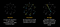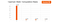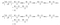Photo by Phil Hearing

# QC — Programming with Quantum Gates (Single Qubits)

Quantum computing uses a different paradigm in writing programs. In this article, we describe the quantum gates — the basic units for quantum programming. If you are not familiar with Qubits, we will suggest you visit Part2 and Part 3 of this series first.

# Qubits manipulation

A superposition can be written as:

# Quantum algorithm

So what is the general flow of a quantum algorithm? First, we prepare the superposition. Then we encode the problem information into the superposition and manipulate it in a high dimensional space. Finally, we apply interference to consolidate the superposition into fewer outcomes. (Note: there are other possibilities and omitted for simplicity.)SourceSource: IBM

# Hadamard Gate

One of the most common quantum gates is the Hadamard Gate.Source

# Global phase

Actually, a superposition can be represented as

# Pauli gates

Other single-qubit gates are pretty straightforward. So feel free to browse through the single-gate quickly. The next 3 Pauli gates rotate the superposition along x, y or z-axis.

# Phase shift

There are a few gates that rotate the vector around the z-axis, namely Z, S, and T-gate.

# Measurement

Here is the symbol in taking a measurement.

# Identity matrix

An identity gate does nothing.

# Next

In general, all these single-qubit operators is about moving the vector along the surface of the unit sphere. Let’s move to multi-qubit gates which are more tricky. These gates deal with entanglement which is extremely important in quantum computing.

Deep Learning